# Selina Concise Mathematics Class 8 ICSE Solutions Chapter 4 Cubes and Cube-Roots

## Selina Concise Mathematics Class 8 ICSE Solutions Chapter 4 Cubes and Cube-Roots (Including use of tables for natural numbers)

Selina Publishers Concise Mathematics Class 8 ICSE Solutions Chapter 4 Cubes and Cube-Roots (Including use of tables for natural numbers)

### Cubes and Cube-Roots Exercise 4A – Selina Concise Mathematics Class 8 ICSE Solutions

Question 1.
Find the cube of :
(i) 7
(ii) 11
(iii) 16
(iv) 23
(v) 31
(vi) 42
(vii) 54
Solution:Question 2.
Find which of the following are perfect cubes :
(i) 243
(ii) 588
(iii) 1331
(iv) 24000
(v) 1728
(vi) 1938
Solution: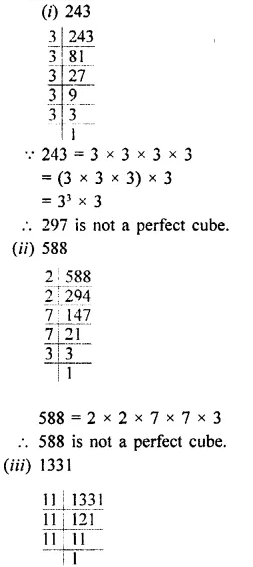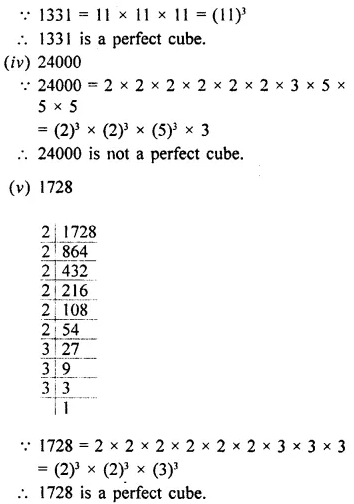Question 3.
Find the cubes of :
(i) 2.1
(ii) 0.4
(iii) 1.6
(iv) 2.5
(v) 0.12
(vi) 0.02
(vii) 0.8
Solution: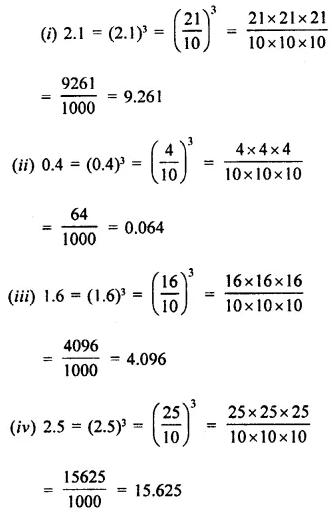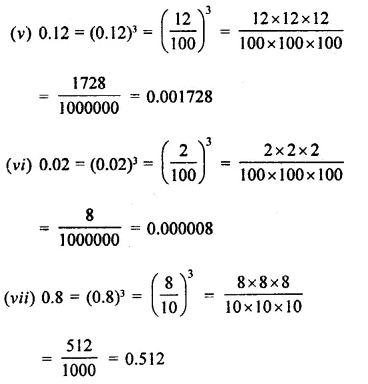Question 4.
Find the cubes of :
(i) $$\frac { 3 }{ 7 }$$
(ii) $$\frac { 8 }{ 9 }$$
(iii) $$\frac { 10 }{ 13 }$$
(iv) $$1\frac { 2 }{ 7 }$$
(v) $$2\frac { 1 }{ 2 }$$
Solution: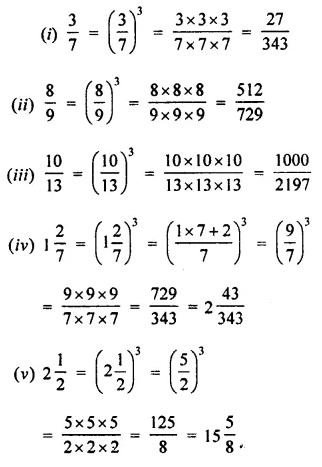Question 5.
Find the cubes of :
(i) -3
(ii) -7
(iii) -12
(iv) -18
(v) -25
(vi) -30
(vii) -50
Solution: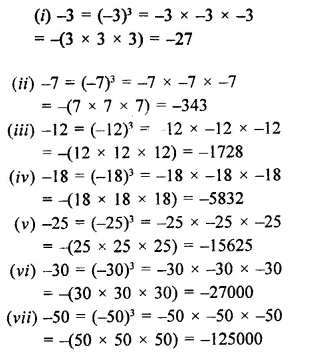Question 6.
Which of the following are cubes of:
(i) an even number
(ii) an odd number
216, 729, 3375, 8000, 125, 343, 4096 and 9261.
Solution: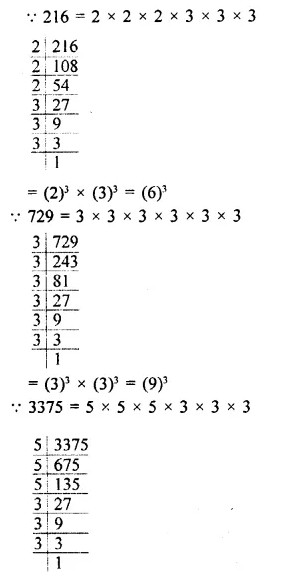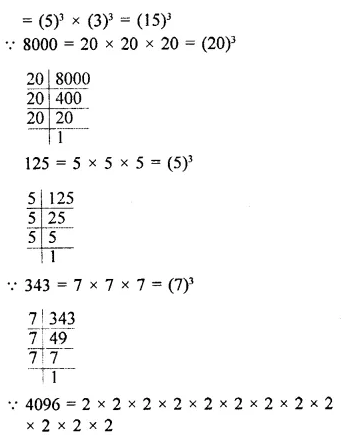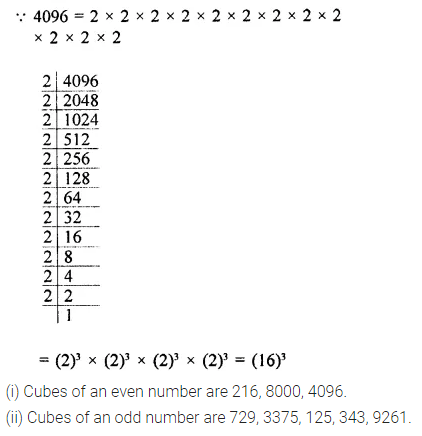Question 7.
Find the least number by which 1323 must be multiplied so that the product is a perfect cube.
Solution: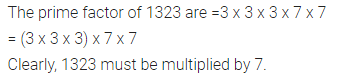Question 8.
Find the smallest number by which 8768 must be divided so that the quotient is a perfect cube.
Solution: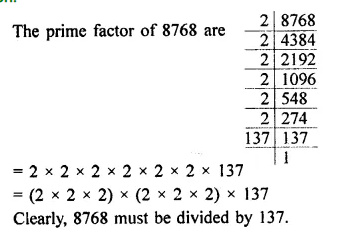Question 9.
Find the smallest number by which 27783 be multiplied to get a perfect square number.
Solution:Question 10.
With what least number must 8640 be divided so that the quotient is a perfect cube?
Solution: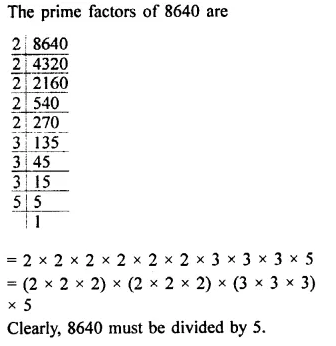Question 11.
Which is the smallest number that must be multiplied to 77175 to make it a perfect cube?
Solution:### Cubes and Cube-Roots Exercise 4B – Selina Concise Mathematics Class 8 ICSE Solutions

Question 1.
Find the cube-roots of :
(i) 64
(ii) 343
(iii) 729
(iv) 1728
(v) 9261
(vi) 4096
(vii) 8000
(viii) 3375
Solution: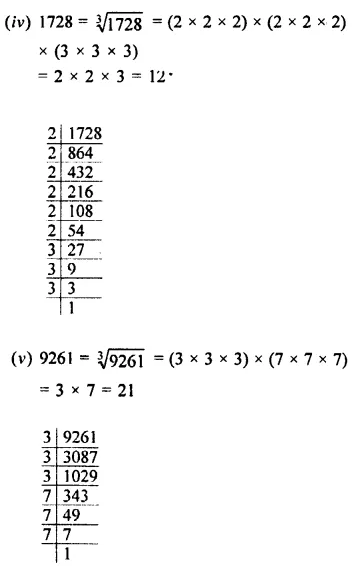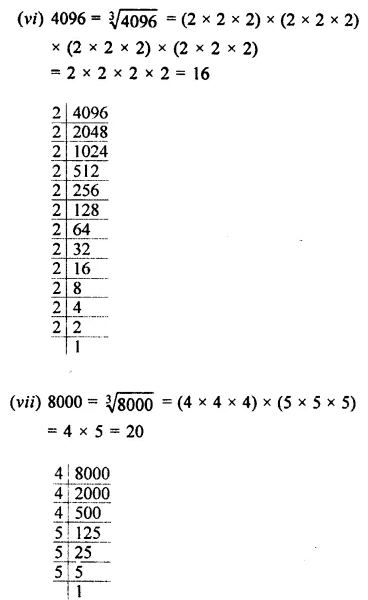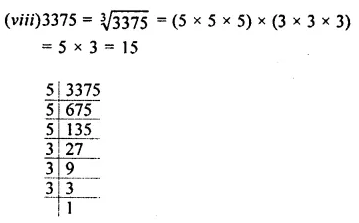Question 2.
Find the cube-roots of :
(i) $$\frac { 27 }{ 64 }$$
(ii) $$\frac { 125 }{ 216 }$$
(iii) $$\frac { 343 }{ 512 }$$
(iv) 64 x 729
(v) 64 x 27
(vi) 729 x 8000
(vii) 3375 x 512
Solution:Question 3.
Find the cube-roots of :
(i) -216
(ii) -512
(iii) -1331
(iv) $$\frac { -27 }{ 125 }$$
(v) $$\frac { -64 }{ 343 }$$
(vi) $$\frac { -512 }{ 343 }$$
(vii) -2197
(viii) -5832
(ix) -2744000
Solution: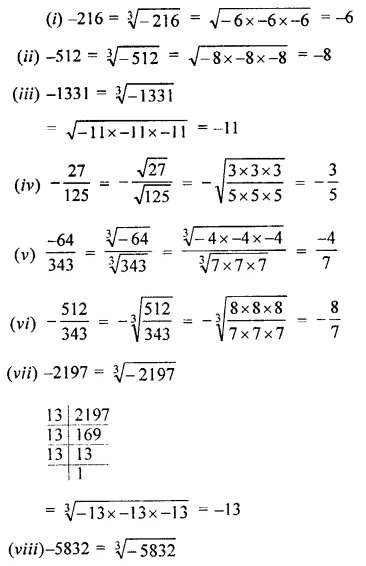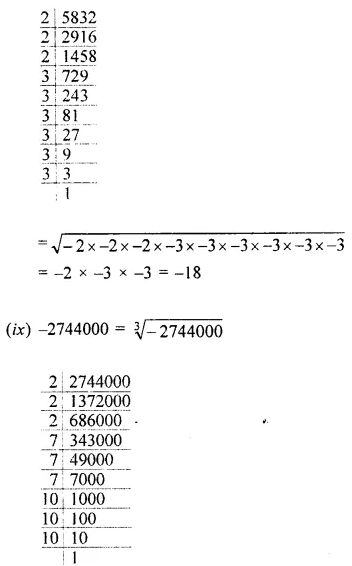Question 4.
Find the cube-roots of :
(i) 2.744
(ii) 9.261
(iii) 0.000027
(iv) -0.512
(v) -15.625
(vi) -125 x 1000
Solution:Question 5.
Find the smallest number by which 26244 may be divided so that the quotient is a perfect cube.
Solution:Question 6.
What is the least number by which 30375 should be multiplied to get a perfect cube?
Solution:Question 7.
Find the cube-roots of :
(i) 700 x 2 x 49 x 5
(ii) -216 x 1728
(iii) -64 x -125
(iv) $$\frac { -27 }{ 343 }$$
(v) $$\frac { 729 }{ -1331 }$$
(vi) 250.047
(vii) -175616
Solution: